Name:    3rd Grade Measurement & DataGeometric measurement: recognize perimeter.  Standard 8, Test 1

Multiple Choice
Identify the choice that best completes the statement or answers the question.

1.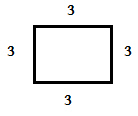What is the perimeter of this polygon?
 a. 9 c. 3 b. 12 d. 15

2.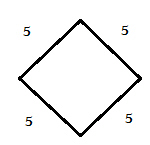What is the perimeter of this polygon?
 a. 20 c. 5 b. 15 d. 10

3.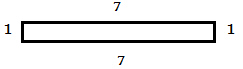What is the perimeter of this polygon?
 a. 51 c. 14 b. 19 d. 16

4.What is the perimeter of this polygon?
 a. 22 c. 20 b. 11 d. 15

5.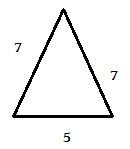What is the perimeter of this polygon?
 a. 14 c. 19 b. 12 d. 16

6.What is the perimeter of this polygon?
 a. 24 c. 21 b. 33 d. 3

7.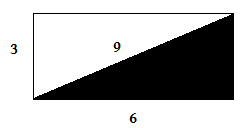What is the perimeter of the white polygon?
 a. 12 c. 15 b. 20 d. 18

8.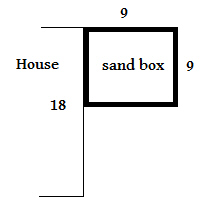Alex decided to build his kids a sandbox in the yard.  What is the perimeter of the sandbox?
 a. 36 c. 27 b. 18 d. 9

9.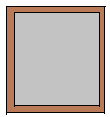Peter was making a cement pad to build a dog house on.  He used 8 foot - 2x4’s to frame out the pad.     What is the perimeter of the pad for the dog house?
 a. 16 c. 30 b. 32 d. 24

10.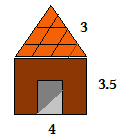The doghouse Peter built looked like this.  What is the perimeter of  the dog house?
 a. 17 c. 10.5 b. 21 d. 20

11.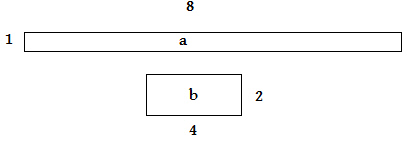Both of these polygons have the same areas.  Which one has the larger perimeter?
 a. a c. they are the same b. b

12.Both of these polygons have the same perimeter.  Which one has the larger area?
 a. a c. they are the same b. b